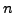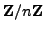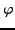# The Ring of Integers ModuloThis chapter is about the ringof integers modulo. First we discuss when linear equations modulohave a solution, then introduce the Eulerfunction and prove Fermat's Little Theorem and Wilson's theorem. Next we prove the Chinese Remainer Theorem, which addresses simultaneous solubility of several linear equations modulo coprime moduli. With these theoretical foundations in place, in Section 2.3 we introduce algorithms for doing interesting computations modulo, including computing large powers quickly, and solving linear equations. We finish with a very brief discussion of finding prime numbers using arithmetic modulo.

Subsections

William 2007-06-01